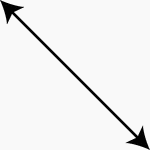### Line, Slanted

A line with arrows on both ends to show that it extends indefinitely. The line is slanting down when…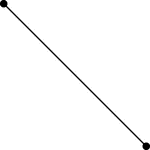### Segment, Slanted Line

Illustration of a slanted line segment. A line segment is a section of a line with definite endpoints.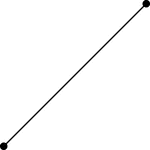### Segment, Slanted Line

Illustration of a slanted line segment. A line segment is a section of a line with definite endpoints.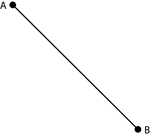### Segment, Slanted Line

Illustration of a slanted line segment. A line segment is a section of a line with definite endpoints.…### Segment, Slanted Line

Illustration of a slanted line segment. A line segment is a section of a line with definite endpoints.…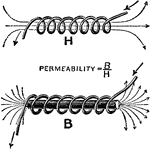### Effect of a metal core in a solenoid

"Illustrating the effects of introducing an iron core into a solenoid. In the upper firgure, the air…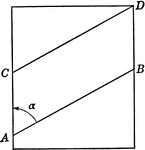### Spiral Drawn on Piece of Paper Shown Flat

Spiral with lines AB and CD drawn on piece of paper shown flat.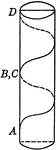### Spiral Drawn on Piece of Paper and Rolled

Spiral with lines AB and CD drawn on piece of paper and rolled into a cylinder to form spiral running…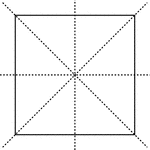### Lines of Symmetry, Square With

Square with dotted vertical, horizontal, and diagonal lines that are lines of symmetry.### Square With Diagonals and Lines Drawn

Illustration showing a square with diagonals, lines, and parallel lines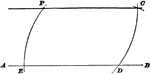### Parallel To Straight Line Construction

Illustration used to show how to draw a straight line parallel to a straight line through a given point.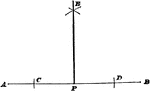### Perpendicular To Straight Line Construction

Illustration used to show how to draw a perpendicular to a straight line from a given point on the line…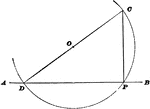### Perpendicular To Straight Line Construction

Illustration used to show how to draw a perpendicular to a straight line from a given point on the line…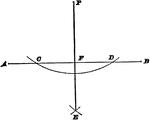### Perpendicular To Straight Line Construction

Illustration used to show how to draw a perpendicular to a straight line from a given point that is…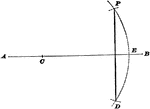### Perpendicular To Straight Line Construction

Illustration used to show how to draw a perpendicular to a straight line from a given point that is…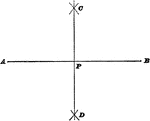### Bisect A Straight Line

Illustration used to show how to bisect a straight line.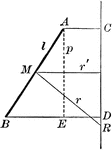### Area of Surface Generated by a Straight Line

Diagram used to prove the theorem: "The area of the surface generated by a straight line revolving about…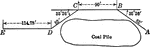### Survey of Lake Front in Chicago

Illustration showing a survey on the lake front in Chicago with distances and angle measures shown.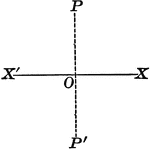### Axis of Symmetry

Illustration of an axis of symmetry drawn with respect to a straight line.### Three Person File Military Formation

An illustration of a three person file military formation.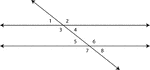### 2 Lines Cut By A Transversal

Illustration of two straight lines cut by a transversal. The 8 angles formed are labeled.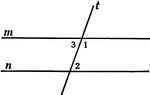### 2 Lines Cut By A Transversal

Illustration of two straight lines, m and n, cut by a transversal t.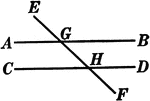### 2 Lines Cut By A Transversal

Illustration of two straight lines, AB and CD, cut by a transversal EF.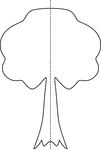### Line of Symmetry, Tree With

Tree with dotted vertical line that shows symmetry.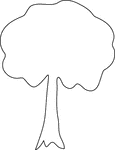### Symmetry, Tree With No

Tree that has no symmetry.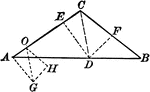### Triangle With Segments

Triangle with segments drawn.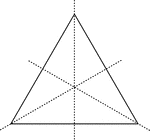### Lines of Symmetry, Triangle With

Square with dotted lines that are lines of symmetry.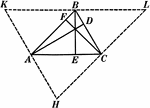### Altitudes In A Triangle

Illustration used to show "The altitudes of a triangle are concurrent."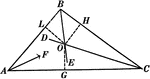### Angle Bisectors In A Triangle

Illustration used to prove "The bisectors of the angles of a triangle are concurrent in a point which…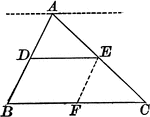### Triangle With Bisector Parallel to the Base

Illustration of a triangle showing that when a line parallel to the base of a triangle bisects one side,…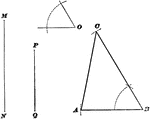### Construction Of Triangle

Illustration used to show how to draw a triangle when given two sides and the included angle of the…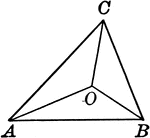### Triangle With Lines From the Vertices That Join a Point Within

Illustration to show lines drawn from the vertices to join at a point within the triangle.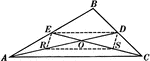### Medians In A Triangle

Illustration used to show "Any two medians of a triangle intersect each other in a trisection point…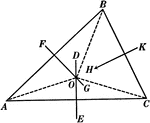### Perpendicular Bisectors In A Triangle

Illustration used to prove "The perpendicular bisectors of the sides of a triangle are concurrent in…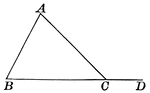### Triangle With Segment Extended for Exterior Angle

Illustration showing a triangle with an exterior segment drawn to show and exterior angle. This can…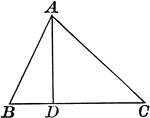### Triangle With Interior Segment

Illustration showing a triangle with an interior segment drawn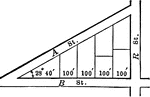### Triangular City Block With Angles and Lengths

Illustration showing an angle of 23 degrees 40 minutes making a triangle in a city block and marking…### Two Person File Military Formation

An illustration of a two person file military formation.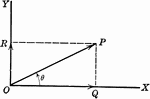### Vector

OP is a line representing a vector (directed quantity)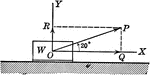### Vector Showing Force at Angle of 20 degrees

OP is a vector (directed quantity) showing the force by a weight acting on it at an angle of 20 degrees.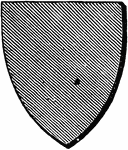### Vert Shield

A heraldic shield with a green (vert) surface, represented by the diagonal lines drawn from the top…### Line, Vertical

A vertical line with arrows at both ends to show that it extends indefinitely.### Line, Vertical

A vertical line with arrows at both ends to show that it extends indefinitely.### Segment, Vertical Line

Illustration of a vertical line segment. A line segment is a section of a line with definite endpoints.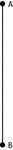### Segment, Vertical Line

Illustration of a vertical line segment. A line segment is a section of a line with definite endpoints.…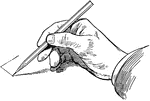### Sketching Vertical Line

Vertical line is drawn downward with the pencil held with freedom, not close to the point.